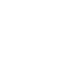# Mar 17 ACT & SAT Question of the Day

If you are connecting to my blog using an email you received from me, do not click the link to sat.collegeboard.org below. Use the link to my website that is farther down on the email.

http://sat.collegeboard.org/practice/sat-question-of-the-day?src=R&questionId=20130317 (This link takes you to today’s question. If you use my archive, you will see the question related to my SAT explanation for that date.)

Here’s wishing you a fine St. Patrick’s Day!

The answer is B. I plugged in numbers, substituting the concrete (actual numbers) for the abstract (a and b).  For answer A, if a=0, then there’s no positive number (the absolute value of b) that I can subtract from 0 that results in 5.  Every answer has to be negative.  B works fine since if a=5, then 5-0 (for b=0), the answer is 5.  C and D result in 0.  For answer E, there’s no positive number that you can subtract from 1 that can give you more than 1.

Changing the abstract to the concrete is a very powerful strategy.  A student told me she used the technique “a lot” on the March SAT.  My count of questions on released tests where the strategy works is about 20%.  It is often much more efficient than doing the math while using variables that you are given.

Let’s see what the ACT folks prepared for us today.

http://www.act.org/qotd/ (The ACT staff does not put a date on their questions so if you click on an archived blog, you’ll get today’s question and the old explanation. Sorry. The SAT staff has dated their questions; so, the archive is helpful. The ACT folks simply don’t do that.)

The answer is A.  Be careful; the question asked “would NOT confirm.” Looking at the chart, you can see if you have a sample with a concentration of a specific amount of anything other than 02 (oxygen),  it will match an entry in the chart for either a depth of 15 or 20.  If it is zero for oxygen, you can’t tell what the depth is.  It could be 10, 15, or 20.

This question is a good example of how to prepare for the ACT Science Test.  Practice reading charts and graphs.  Work on your speed.  Don’t waste time reviewing science topics; you couldn’t possibly figure out what to review since the science knowledge that shows up on the test is very unpredictable.